# Lucas polynomials

(diff) ← Older revision | Latest revision (diff) | Newer revision → (diff)
Jump to: navigation, search

The polynomials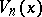(cf. [a1] and [a5]) given by(a1)

They reduce to the Lucas numbersfor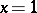, and they satisfy several identities, which may be easily proved by induction, e.g.: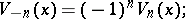(a2)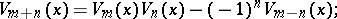(a3)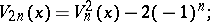(a4)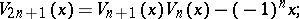(a5)(a6)

wheredenote the Fibonacci polynomials;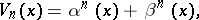(a7)

where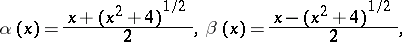so that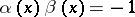; and(a8)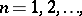where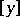denotes the greatest integer in.

The Lucas polynomials are related to the Chebyshev polynomials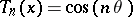,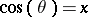, by(a9)

J. Riordan [a9] considered the polynomials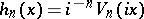and the Lucas-type polynomials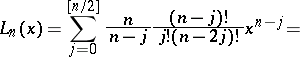(a10)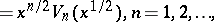in a derivation of Chebyshev-type pairs of inverse relations. V.E. Hoggatt Jr. and M. Bicknell [a4] found the roots of. These are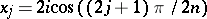,. Bicknell [a2] showed that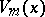dividesif and only ifis an odd multiple of. G.E. Bergum and Hoggatt Jr. introduced in [a1] the bivariate Lucas polynomials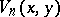by the recursion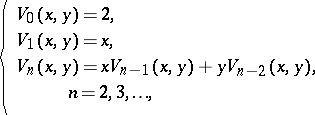(a11)

generalized (a7) for, and showed that theare irreducible polynomials over the rational numbers if and only iffor some positive integer (cf. also Irreducible polynomial). The formula(a12)which may be derived by induction onor by expanding the generating function of, generalizes (a8).

Ch.A. Charalambides [a3] introduced and studied the Lucas and Lucas-type polynomials of order,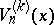and. The Lucas-type polynomials of ordersatisfy the recurrence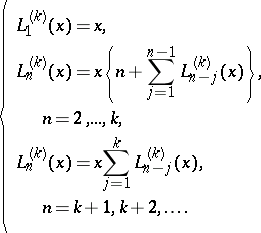(a13)

These polynomials have the binomial and multinomial expansions(a14)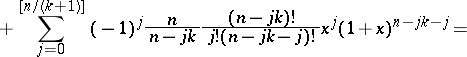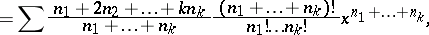where the second summation is taken over all non-negative integers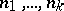such that, and they are related to the Fibonacci-type polynomials of order(cf. [a6] and [a8] and Fibonacci polynomials),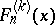, by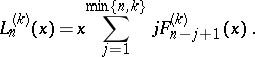(a15)

Furthermore,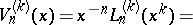(a16)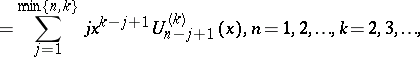where theare the Fibonacci polynomials of order(cf. [a7]). Charalambides [a3] showed that the reliability of a circular consecutive-out-of-:-system,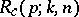, whose components function independently with probabilityis given by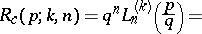(a17)How to Cite This Entry:
Lucas polynomials. Encyclopedia of Mathematics. URL: http://encyclopediaofmath.org/index.php?title=Lucas_polynomials&oldid=17297
This article was adapted from an original article by Andreas N. Philippou (originator), which appeared in Encyclopedia of Mathematics - ISBN 1402006098. See original article